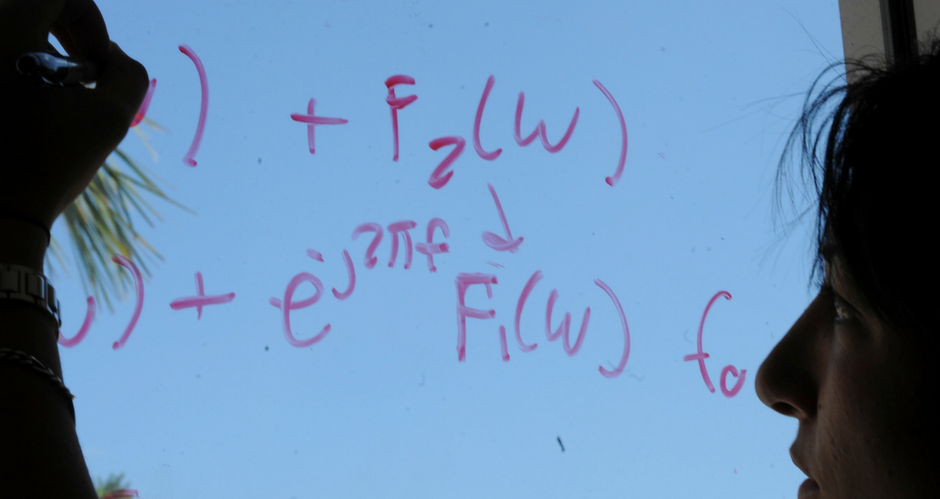## Mathematics and System Engineering Faculty Publications

### Dini-Campanato spaces and applications to nonlinear elliptic equations

Article

#### Publication Title

Electronic Journal of Differential Equations

#### Abstract

We generalize a result due to Campanato [C] and use this to obtain regularity results for classical solutions of fully nonlinear elliptic equations. We demonstrate this technique in two settings. First, in the simplest setting of Poisson's equation Delta u=f in B, where f is Dini continuous in B, we obtain known estimates on the modulus of continuity of second derivatives D2u in a way that does not depend on either differentiating the equation or appealing to integral representations of solutions. Second, we use this result in the concave, fully nonlinear setting F(D^2u,x)=f(x) to obtain estimates on the modulus of continuity of D^2u when the L^n averages of f satisfy the Dini condition.

1

20

9-25-1999

COinS